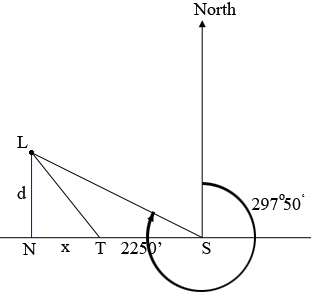SEARCH HOMEMath Central Quandaries & QueriesQuestion from Kirby, a student: A ship is sailing due west when a light is observed with heading 297°50' from the ship. After the ship has travelled 2250 feet, the light heads 311°55' from the ship. If the course is continued, how close will the ship approach the light?Hi Kirby,

In my diagram $S$ is the initial position of the ship, $T$ is its position after it has travelled 2250 feet, $L$ is the position of the light and $N$ is the closest point to $L$ on the path being followed by the ship. The angle $LNS$ is a right angle.Bearing is measured clockwise from north so in my diagram the angle $NSL$ has measure $297^0 50^' - 270^{o}.$ What is the measure of the angle $NTL?$ Use trigonometry and the right triangles $LNT$ and $LNS$ to find two equations in the variables $x$ and $d.$ Solve for $d.$

PennyMath Central is supported by the University of Regina and the Imperial Oil Foundation.